Return to Free Library

Return to Number Menu

We will now move into a two-part series on the Heptad and music.

# Goethe tells us, “Geometry is frozen music.”

“Music, in the absolute sense, is the invisible geometry of the cosmos, a delicate tracery of frequencies that harmonize with each other and from which all matter manifests.

Music, as sensed by humans, is a delicate tracery of audible frequencies that harmonize with each other and generally please our emotions.”1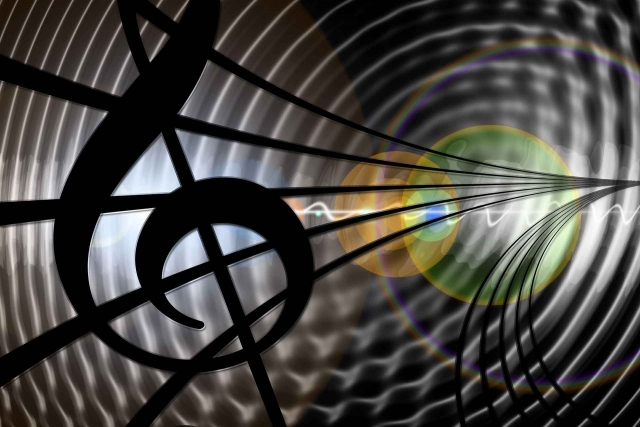# Audible Range – The Octaves of Human Hearing

We hear approximately 10 octaves of sound.

Every frequency in the audible range is a note.

The scale begins at 16 cycles per second (16 Hertz) and increases exponentially to around 20,000 Hz.The highest note on a piano is 4186 Hz.

Below 16 Hz we experience rhythm.

# Music

Music is the study of the proportional laws of sound frequency.  These proportional laws are related to harmony.  Thus, music is harmony of sound.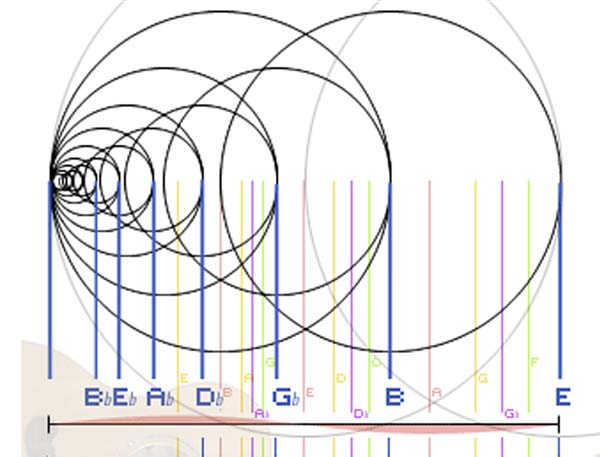The proportional ‘laws’ of music.

The word “music” is from the Greek mousike “muselike”.

It derives from the Egyptian glyph mes meaning “birth” or “generation”.  The ancient Egyptians recognized that wave frequencies create all in physical reality.

There are agreeable sounds and disagreeable sounds due to tones being combined harmoniously or discordantly.

Music has been used throughout time to bypass the intellect and appeal directly to the passions and emotions.# Music & Reciprocity

Changes in frequency & wavelength are reciprocal.

The greater the energy (the higher the frequency) the shorter the wavelength.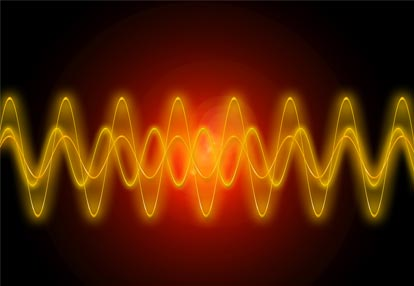Rising or falling tones, as reciprocal arithmetic ratios, are applied to string lengths.

There are ‘Major’ and ‘Minor’ reciprocal tonal patterns.

# Notes (Tones)

A note is a sound with fundamental frequency.  It is usually accompanied by a number of harmonics.  These ‘harmonics’ are embedded in the note and are a fact of nature.

The fundamental frequency identifies the note.

Every frequency in the audible range is a note.

A Chord is a summation or grouping of notes sounded simultaneously.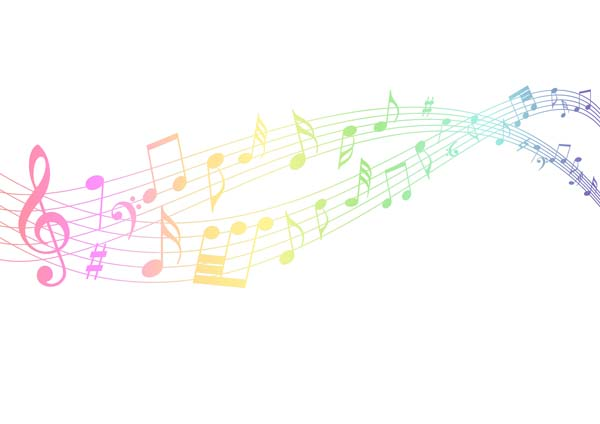# Scale

The audible range is divided into discrete steps.

A scale is a series of steps.

Many scales have been created.

The most common scale is the modern even-tempered Western scale.  This divides the octave into 12 equal steps.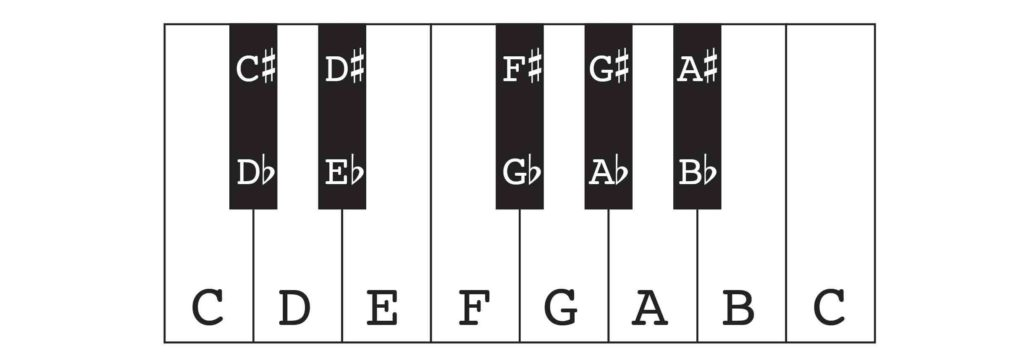Other scales are as follows:

• Pentatonic – 5 notes (6th as octave)
• Diatonic – 7 notes (8th as octave)
• Chromatic – 12 notes (13th as octave)
• Shruti – 21 notes (22nd as octave)

“Looking at the pattern of the keyboard,” Gyorgy Doczi tells us, “we recognize its harmonious, golden proportions: there are 8 white keys, there are 5 black keys, and these black keys are in groups of 2’s and 3’s.  The series 2:3:5:8 is, of course, the beginning of the Fibonacci series.”

# Overtones – Harmonics

Harmonics are defined as “a musical tone that is a part of the harmonic series above a fundamental note and may be heard with it”.  These are based on proportions expressible in the smallest whole numbers: 1, 2, 3, 4.

“Most oscillators, from a guitar string to a flute, will naturally vibrate at a series of distinct frequencies known as normal modes. The lowest normal mode frequency is known as the fundamental frequency, while the higher frequencies are called overtones.”2

“We find the 1:2, 2:3 and 3:4 proportions reappearing in the first and strongest overtones, also called partials or harmonics, which reverberate within every musical sound, blending with its fundamental tone, as if additional, invisible strings were being plucked simultaneously, accompanying and complementing the fundamental.” ~ Gyorgy Doczi – The Power of Limits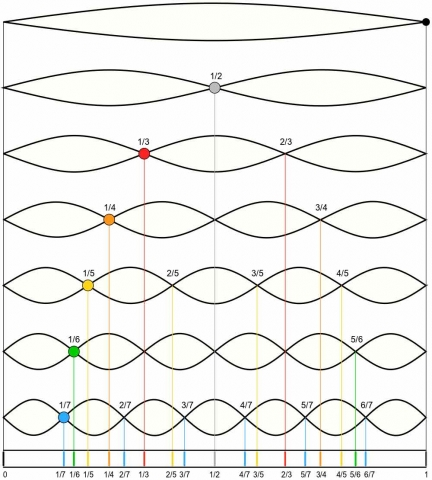The harmonic series chart.  Each harmonic is embedded into each fundamental note.

When an oscillator (ex. Guitar string) is excited it will oscillate at several of its modal frequencies at once.

This gives the sensation of hearing other frequencies (overtones) above the lowest frequency (the fundamental or tonic).

Ideal overtones (harmonic partials) are integer multiples of the fundamental.  It is based on mathematical proportions discussed below.

# Consonance & Dissonance

Consonance and dissonance are subjective labels that have evolved over time.

Consonance – the pleasing, relaxing qualities of certain chords

It evokes a feeling of stability.Dissonance – the displeasing, tension-causing qualities of certain chords

This evokes a feeling of tension and movement.According to Helmholtz Theory the degree of dissonance among a group of notes is determined by the number of strong harmonics that are close but not equal.

When two notes are close but not equal and they sound simultaneously they cause a pulsing called ‘beating’.

If a chord has many ‘beats’ it creates a rough sound, unpleasant to hear.

# Beat Frequencies

When two frequencies are sounded simultaneously, a third frequency is created called the ‘beat frequency’.

It is equal to the difference between the two original frequencies.  It is a mathematical proportion.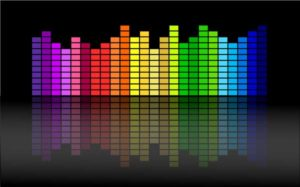# Binaural Beat Frequencies

When two frequencies are sounded simultaneously, one in each ear, the brain produces an ‘imaginary’ beat frequency.

When the beat frequency fits achievable brainwave frequencies, the brain waves of the listener tend to entrain to that beat frequency.

Binaural beating can be used to direct brain wave states.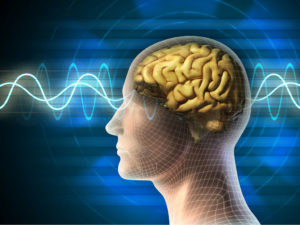# Harmony

Harmony comes from harmonia, meaning “fitting together”.

Harmony “comes from the reconciliation of opposites by a third element, bringing them all a new unity.”3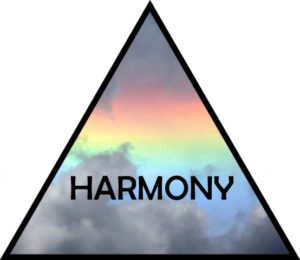Harmony naturally appears on the string when it is divided into unequal parts.

It is related to the simultaneous coincidence of additive and multiplicative processes – just as seen in the square and the proportion φ (phi).

Harmony is a healthy body and mind.  This refers to the rhythmic relationships which accompany and define the state of vibrant health on all levels.# Harmonic Resonance in a Cavity

Air within a cavity (enclosed space) undergoes a special type of vibration due to the enclosure.

As sound waves bounce around standing waves develop.

Standing waves do not travel; they define a geometric energy distribution.

The energy distribution is composed on a series of precisely related resonant frequencies called harmonics or modes.

The series of harmonics within a resonant cavity is precisely defined by the geometry of the cavity.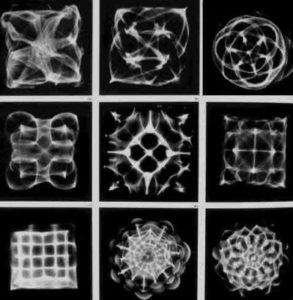Cymatics Patterns.  Sound (Vibration) creates geometry.

“The harmonic series of a resonator is a unique signature for the resonator’s geometry. For instance, a chemical molecule can be precisely identified by the frequencies present in the light it emits. In the same way, a resonant cavity is precisely identified by the frequencies present in its resonant harmonic series, as well as their relative proportions.”4

# Ratios

We have discussed ratios several times before in Cosmic Core.  Ratios refer to the mathematical ratio between two notes.  This is demonstrated through Mediation, discussed below.

Reference Construction Lesson #72: Geometry & Music.

# Mediation

Mediation is the binding of two extremes by a single mean term, or

the binding of two “opposite” tones by harmoniously placing a third tone between them.

We respond not to the tones themselves, but to their relationship – their musical interval.The relationship between tones – their musical interval.

# Mediation and Proportion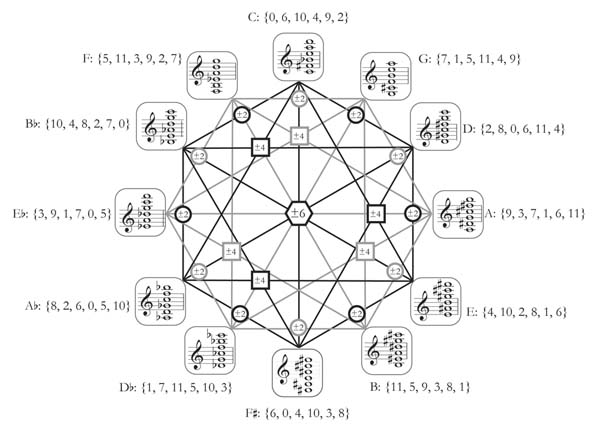Mediating Proportions are based upon triads or threes.

A mediating proportion can be defined as ‘A group of three unequal numbers such that two of their differences are to each other in the same relationship as one of these numbers is to itself or to one of the other two numbers’.

We will now break this statement down to better understand what it means.

A median proportion is formed from a group of any three numbers with a greater than b and b greater than c (a > b > c), such that “two of their differences”, that is:

a – b (this is one difference)

b – c (this is the second difference)

“…are to each other” :   a – b : b – c

“…in the same way as one of these numbers is to itself”

(Case 1)           a – b : b – c : : a : a      or

a – b : b – c : : b : b      or

a – b : b – c : : c : c

“…or as one of these numbers is to one of the other numbers”:

(Case 2) a – b : b – c : : a : b   or

(Case 3) a – b : b – c : : a : c

## There are three important proportions or medians that we will discuss now:

• Arithmetic Proportion (Case 1)
• Geometric Proportion (Case 2)
• Harmonic Proportion (Case 3)

# Three Major Medians

## Arithmetic Proportion (Case 1)

Arithmetic:  b = (a + c)/2

3, 5, 7 is an arithmetic progression with arithmetic mean, b = 5.

This contains the law for addition and its inverse subtraction.

It describes the relationship which gives the natural series of cardinal numbers, 1, 2, 3, 4, 5, 6…etc.

It shows an equality of difference, but inequality of ratio.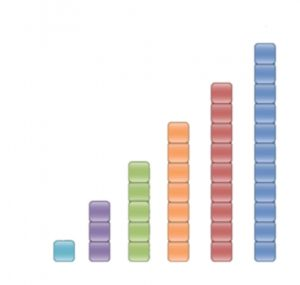Arithmetic Progression.  Equality of difference (increases by 2 each time).  Inequality of ratio (1:3 does not equal 3:5 or 5:7…etc).

## Geometric Proportion (Case 2)

Geometric:  b2 = ac

4, 8, 16 is a geometrical progression with geometric mean, b = 8.

This contains the law for multiplication and its inversion, division.

It describes the relationship which gives any series of geometric progression.

It shows equality of ratio but inequality of difference.

It is called the ‘perfect proportion’ because it is a direct proportional relationship, an equality of proportion bound by one mean term.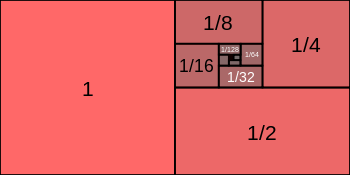Geometric Progression.  Equality of ratio (1:1/2 = 1/2 :1/4…etc).  Inequality of difference (increases by 1/2 then 1/4…etc).

## Harmonic Proportion (Case 3)

Harmonic:  b = (2ac)/(a+c)

2, 3, 6 is a harmonic progression with harmonic mean, b = 3.

3 = 24/8 = (2 x 2 x 6)/2+6

This proportion is derived from a combination of the first two.

It is formed by a multiplication of any two extremes (a, c) followed by the division of this product by their average or arithmetic mean (a + c)/2.

Another example: given two extremes (a, c) = 6 and 12

The products of 6 and 12 = 72

The arithmetic mean between 6 and 12 = 9

72/9 = 8

So: 6, 8, 12 is a harmonic progression.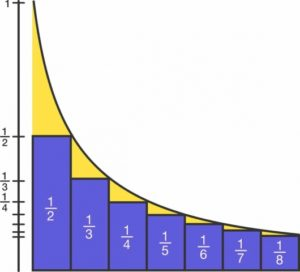Harmonic Progression

The inverse of every harmonic progression is an arithmetic progression.

Example: 2, 3, 4, 5 = ascending arithmetic progression

½, 1/3, ¼, 1/5 = descending harmonic progression

# The Musical Proportion – 1, 4/3, 3/2, 2

Gyorgy Doczi tells us, “Two strings sound most pleasant together when they are equal, or when one is plucked at ½, 2/3, or ¾ of the other’s length; in other words, when the length of the plucked strings relate in proportions expressible in the smallest whole numbers: 1, 2, 3, 4.”

The insertion of the harmonic and arithmetic means between the two extremes in double ratios (representing the double octave) gives the ‘musical’ proportion 1, 4/3, 3/2, 2.

“In other words, the arithmetic and harmonic means between the double geometric ratios are the numerical ratios which correspond to the tonal intervals of the perfect fourth and the perfect fifth, the basic consonances in nearly all musical scales.5An example of an octave of notes.  A is the ‘fifth’ of C.  G is the ‘fourth’ of C.

The next article will explain the fourth, fifth and octave in greater detail and clarity.

For now, know that:  1, 4/3, 3/2, 2 represents the frequencies of a fundamental fourth (4/3), fifth (3/2) and octave (2/1).

“The 1:1 proportion, which is identity, is called unison,” explains Gyorgy Doczi.  “The 1:2 proportion is called the octave.  The pleasant sound of the 2:3 proportion was called diapente (penta, “five”) today called the fifth, reaching through five intervals.  The consonance of the 3:4 proportion was called diatessaron (tessares, “four”) or the fourth.”

Using strength length = 1      (Note C)

Dividing the string in half = 1:2 (the octave)       (Note C one octave up)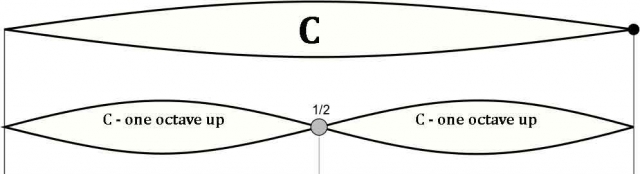Harmonic mean between 1 and ½ = 2/3 (the fifth 3:2) – the string is divided into thirds.  It is called ‘the fifth’ because it is the fifth note up (Note A) from the first note (the original C).  This means that sounding 1/3 of the string produces the fifth note ahead in the musical scale.

Arithmetic mean between 1 and ½ = ¾ (the fourth 4:3) – the string is divided into fourths.  It is called ‘the fourth’ because it is the fourth note up (Note G) from the first note C.  This means that sounding 1/4 of the string produces the fourth note in the musical scale.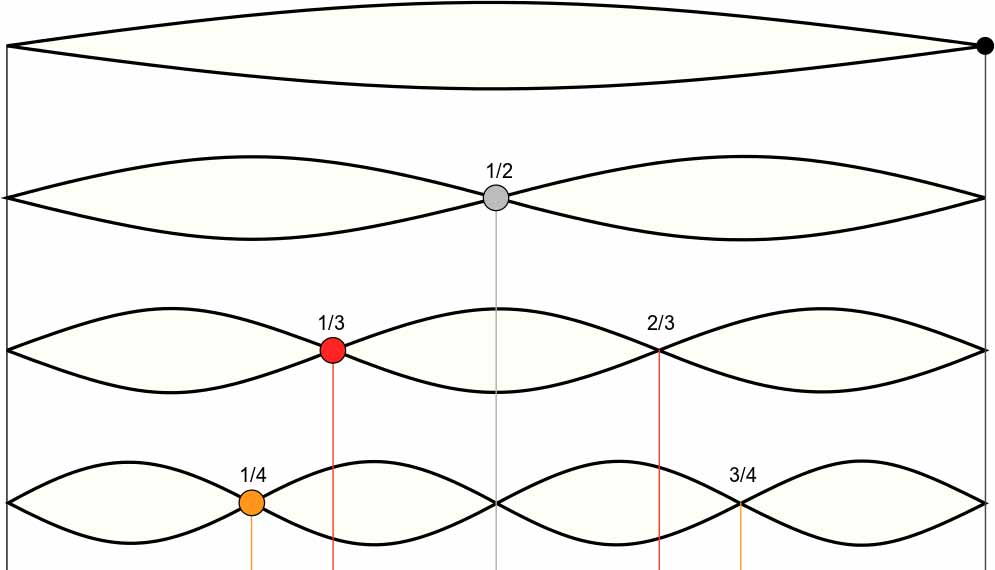This illustrates the whole note, the octave, the fifth and the fourth.

“We see an inversion of ratios and a crossing of functional positions between the arithmetic and harmonic mean.”6

Musical harmony develops out of a simultaneous inversion, as seen above.

Musical harmony also contains simultaneity of addition and multiplication.

The fifth + the fourth = the octave (4/3  x  3/2 = 2).

The combined effect of addition and multiplication produces the logarithm in mathematics.  The Golden Proportion (φ) is the archetype for this form of growth.

Gyorgy Doczi in The Power of Limits, explains the relationships between the golden section and the minor and major modes of the Western scale:  “The two parts of the golden section’s proportions are unequal:  one is smaller, the other larger.  They are often referred to as minor and major.  Minor and major here are opposites united in a harmonious proportion.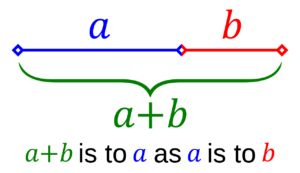Our Western diatonic scales and chords are yet further instances of the 1:2, 2:3, and 3:4 proportions in the dinergy of musical harmonies.  The two chief modes of Western scales, the minor (considered to be the sad one) and the major (associated with brightness) differ from each other only in the length of steps between certain of their intervals, just as the minor and major parts of the golden section differ from each other only in their lengths.  And just as the joining of minor and major parts delights us in the visual harmonies of the golden section, so the joining of minor and major scales, called modulations, enchant us when we hear them in the movement of chords and melodies.”

So,”The 2:3 = 0.666 proportion of diapente is a close approximation of the golden section’s 0.618…ratio.  Diatessaron is identical with the 3:4 proportion of the Pythagorean triangle.  Daipason, the octave, has the 1:2 = 0.5 proportion of a rectangle composed of two equal squares, having a diagonal of √5 length, which is the joint length of two reciprocal golden rectangles.”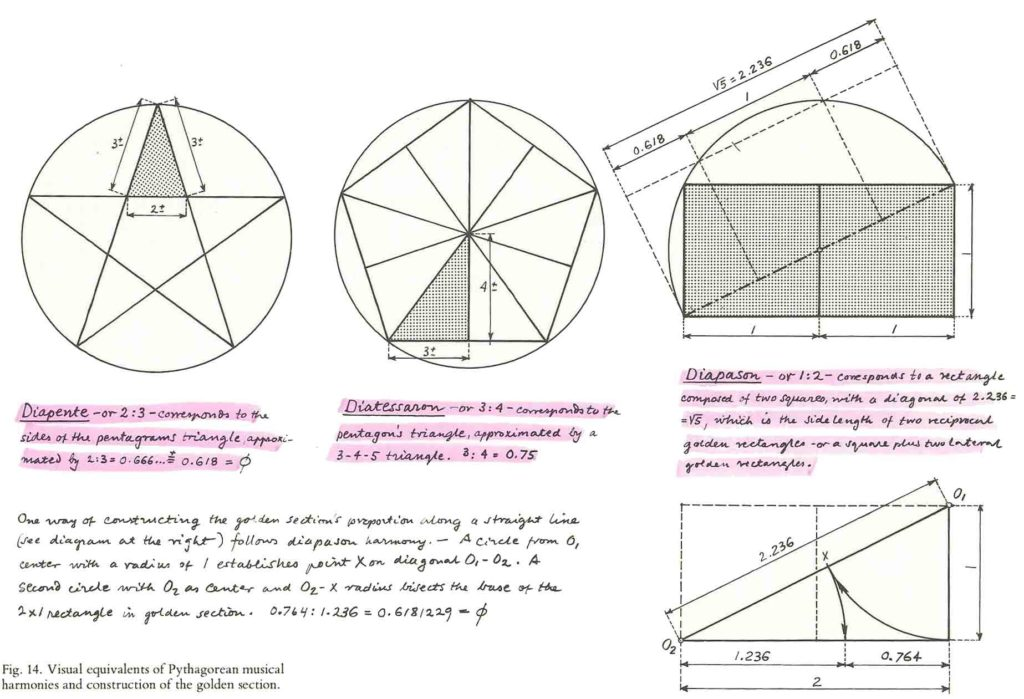Credit: Gyorgy Doczi – The Power of Limits, 1981

To recap: Addition and multiplication combined produce the logarithm.  A logarithm is “a quantity representing the power to which a fixed number (the base) must be raised to produce a given number”.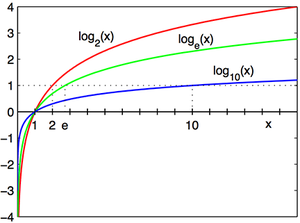Examples of logarithmic growth.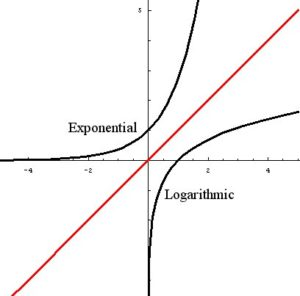The difference between exponential and logarithmic growth.Therefore:  The harmonic progression is a sequence of real numbers formed by taking the reciprocals of an arithmetic progression. Equivalently, it is a sequence of real numbers such that any term in the sequence is the harmonic mean of its two neighbors.

# Healing Music

“Cosmic harmonies played with earthly strings bring the archetypal principles to earth, thus making music therapeutic.”7Understanding the physics of sound empowers one to use sound with clarity, direction and confidence.

Intention is key.

Love is the power.Healing music has been used in many cultures and times:

• Egyptian, Babylonian, Indian, Chinese, African, Native American, Persian
• Mythical Apollo (Greek god of harmony) – involved with music, poetry, prophecy, medicine, art and archery• Orpheus received a lyre from Apollo and used it guide his wife Eurydice from the darkness of the underworld into the light.# Psychological Response to Various Sounds

Simple harmonic ratios are usually considered concordant, and promote a sense of contentment.

Sounds with very strong upper harmonics promote a sense of dis-ease (screeching of breaks; nails on a chalkboard).

Low inaudible frequencies (1-15Hz) often include a sense of impending danger or wariness (these frequencies usually accompany earthquakes and explosions).

Sharp, quick sounds are psychologically invigorating.

Smooth and soft sounds are psychologically soothing.# Entrainment

Entrainment is fundamental to understanding the use of sound for positive and therapeutic psycho-physiological effects.

Entrainment is resonance in the special context of coupled systems tending toward synchronization.

Coupled systems are two systems energetically or informationally dependent.

Most system behavior is dependent on many systems, each of these also dependent on many others.

All systems are vibrational and interact by means of vibrational signals.

Coupled systems tend towards resonance when they share natural resonant frequencies.

Resonance leads to the tendency towards synchronization, that is, entrainment.So, systems tend towards entrainment:

• when the function of each system is sufficiently dependent on the function of the other
• if they possess natural resonant frequencies which are sufficiently close

If two systems share harmonics, but not fundamentals they also tend toward entrainment, though not as powerfully.

Synchronization is the lowest energy state in systems of sufficiently identical coupled oscillators.

Hamilton’s principle – all mechanical systems tend toward the lowest energy state.

Systems with sufficiently similar natural resonant frequencies and sufficiently strong coupling naturally tend to entrain.

# Entrainment & the ‘Center of Rhythm’

“Entraining rhythmic systems tend toward a sort of ‘center of rhythm’ which is closer to the behavior of the stronger system.”8

There is a metaphysical component – stronger systems can be seen as more coherent systems.  Coherent systems involve harmony and are those thoughts, feelings, emotions, actions that involve order – that is those of love, compassion, peace, health and empathy.

This then means that the ‘positive’ is stronger than the ‘negative’ as the positive brings order (unity) and the negative brings chaos (separation).

That which coheres grows stronger.  That which separates, eventually falls apart and disappears.1. http://www.cymascope.com/
2. https://en.wikipedia.org/wiki/Harmonic
3. Schneider, Michael, A Beginner’s Guide to Constructing the Universe, Harper Perennial, 1994
4. Anderson, Thomas, Sound Therapy Foundations: They Physics of Sound and Vibration, November 2012
5. Lawlor, Robert, Sacred Geometry: Philosophy & Practice, Thames & Hudson, 1982
6. ibid.
7. Schneider, Michael, A Beginner’s Guide to Constructing the Universe, Harper Perennial, 1994
8. Anderson, Thomas, Sound Therapy Foundations: They Physics of Sound and Vibration, November 2012

Return to Free Library

Return to Number Menu